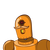# WORKSHEET-1Fill in the blanks 10 X 1=10M1. The expanded notation for 10,805=l2. Make the smallest 5-Digit number

WORKSHEET-1
Fill in the blanks 10 X 1=10M
1. The expanded notation for 10,805=l
2. Make the smallest 5-Digit number by using
1,5,3,9,2
3. 83,76,300=
+ 300000 +70000
4. Make the greatest 6- Digit number by using
2,9,0,1,8,3
5. The place value of digit 8 in 8,12,565 ?

### 1 thought on “WORKSHEET-1<br />Fill in the blanks 10 X 1=10M<br />1. The expanded notation for 10,805=l<br />2. Make the smallest 5-Digit number”

1.1. 1.0805 × 10⁴

2. 12,359

3. 83,76,300=83,39,300

+ 300000 +70000

4. 9,83,210

5. lakhs

Step-by-step explanation: